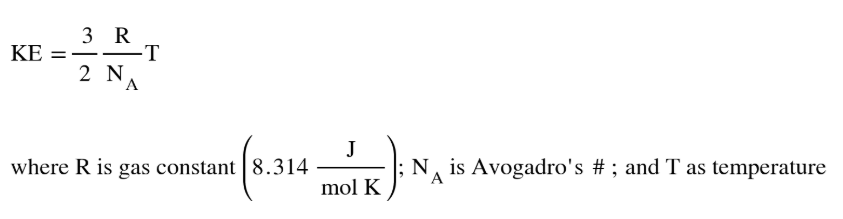Chemistry Practice Problems Kinetic Energy of Gases Practice Problems Solution: Calculate the average kinetic energies of CH4(g) a...

🤓 Based on our data, we think this question is relevant for Professor Griffith's class at UMD.

# Solution: Calculate the average kinetic energies of CH4(g) and N2(g) molecules at 273 K and 546 K.

###### Problem

Calculate the average kinetic energies of CH4(g) and N2(g) molecules at 273 K and 546 K.

###### Solution
• Average kinetic energy of gases, which is derived from the RMS (root mean square speed) and KE equation, can be solved as:• It appears that the average KE of gases is dependent on the temperature which means that all gases will have the same KE as long the temperature is the sameView Complete Written Solution

Kinetic Energy of Gases

Kinetic Energy of Gases

#### Q. Calculate the kinetic energy, in joules of a 1260-kg automobile moving at 18.0 m/s.

Solved • Thu Oct 25 2018 15:11:39 GMT-0400 (EDT)

Kinetic Energy of Gases

#### Q. A baseball weighs 5.13 oz. What is the kinetic energy, in joules, of this baseball when it is thrown by a major league pitcher at 95.0 mi/h?

Solved • Thu Oct 25 2018 15:11:38 GMT-0400 (EDT)

Kinetic Energy of Gases

#### Q. Consider a 1.0-L container of neon gas at STP. Will the average kinetic energy, average velocity, and frequency of collisions of gas molecules with th...

Solved • Thu Oct 18 2018 10:59:36 GMT-0400 (EDT)

Kinetic Energy of Gases

#### Q. A 100.-L flask contains a mixture of methane (CH4) and argon gases at 25°C. The mass of argon present is 228 g and the mole fraction of methane in the...

Solved • Thu Oct 18 2018 10:41:34 GMT-0400 (EDT)